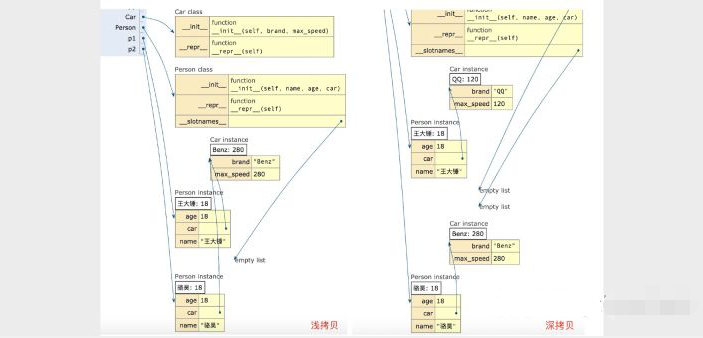# 再论深拷贝和浅拷贝

class Person:

def __init__(self, name, age):
self.name = name
self.age = age

def eat(self, food_name):
print(f'{self.name}正在吃{food_name}.')

def __repr__(self):
return f'{self.name}: {self.age}.'

p1 = Person('王大锤', 18)
p2 = p1


import copy

p1 = Person('王大锤', 18)
p2 = copy.copy(p1)


p1 == p2            # False
p2.name = '骆昊'
p2.age = 39
print(p1, p2)       # 王大锤: 18. 骆昊: 39.


import copy

class Car:

def __init__(self, brand, max_speed):
self.brand = brand
self.max_speed = max_speed

def __repr__(self):
return f'{self.brand}: {self.max_speed}'

class Person:

def __init__(self, name, age, car):
self.name = name
self.age = age
self.car = car

def __repr__(self):
return f'{self.name}: {self.age}'

p1 = Person('王大锤', 18, Car('QQ', 120))
p2 = copy.copy(p1)
p2.name = '骆昊'
p2.car.brand = 'Benz'
p2.car.max_speed = 280
print(p1, p1.car)
print(p2, p2.car)import copy

class PrototypeMeta(type):

def __init__(cls, *args, **kwargs):
super().__init__(*args, **kwargs)
cls.clone = lambda self, is_deep=True: \
copy.deepcopy(self) if is_deep else copy.copy(self)

class Car:

def __init__(self, brand, max_speed):
self.brand = brand
self.max_speed = max_speed

def __repr__(self):
return f'{self.brand}: {self.max_speed}'

class Person(metaclass=PrototypeMeta):

def __init__(self, name, age, car):
self.name = name
self.age = age
self.car = car

def __repr__(self):
return f'{self.name}: {self.age}'

p1 = Person('王大锤', 18, Car('QQ', 120))
p2 = p1.clone(is_deep=True)    # 深拷贝
p3 = p1.clone(is_deep=False)   # 浅拷贝